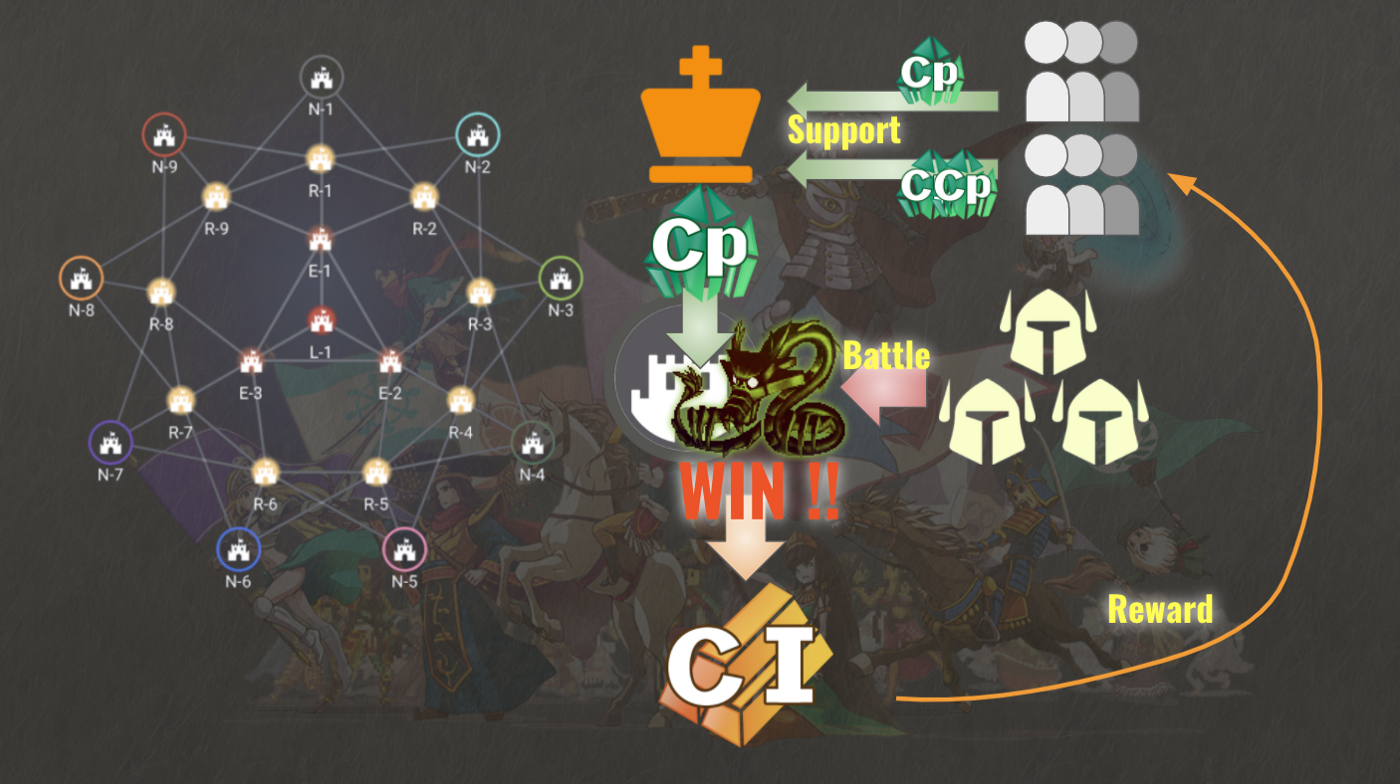# Help

Back to the list

## Reward for Cryptid Wars

### Main Reward[2021.03.29] There is a change in the formula for calculating ingots from Season 28. The help below has been updated to the latest version.

In future iterations, GenEx, used to create Land Extensions, and Cp（Cryptonium） will be given as rewards.

### How many CI you can get

In Cryptid Wars, you can earn CI based on your Cp consumption. They will be calculated and distributed as follows at the end of the season.

• From the Cp consumed by Rand in Cryptid Wars, the amount of CI distributed will be calculated according to the current conversion rate and the percentage of fort points earned.

※[ Rand consumed Cp * Fort Point Rate (below) / Conversion Rate ]

Fort Point Rate: Calculate the ratio of your land's fort points when the total fort points of the land is 100%. Multiply it by 10. The average is 110%. It goes up and down depending on the contribution of the rand.

※Conversion Rate：Calculated as follows.

[Max supply except staking reward (45 million) / Remaining supply * 200]

### Raking Bonus

Depending on the rank of the rand, a bonus Cp is awarded and can be obtained as ingots.

[ Bonus Cp for Rand Rank (below) / Conversion Rate ]

• 1st: 13 million Cp

• 2nd: 10.5 million Cp

• 3rd: 8 million Cp

• 4th: 6 million Cp

• 5th: 4.5 million Cp

• 6th: 3.5 million Cp

• 7th: 2.5 million Cp

• 8th: 1.5 million Cp

• 9th: 0.5 million Cp

The CI which is distributed to each citizen within each Land on a CI rate in proportion to what was contributed.

＞How to get Crypt Ingots (CI)

Back to the list